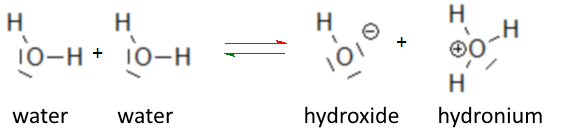Search:

# The ionic product of water

Applying the law of mass action to the autoprotolysis equilibrium of water:it is found for any solution containing these three species: $\frac{[OH^-][H_3O^+]}{[H_2O]}$ $=$ $K$ $[OH^-][H_3O^+]$ $=$ $K[H_2O]$ The equilibrium constant $K$ depends only on the temperature and as long as we limit ourselves to low concentrations of other species present in the aqueous solution, $H_2O$ remains approximately constant, hence: $[OH^-][H_3O^+]$ $=$ $K_e$ Under these conditions $K_e$ is constant (valid for any dilute solution at a given temperature and also of course for pure water). The values $[OH^-]$=$[H_3O^+]$=$10^{-7}$ valid for pure water at 25oC allow to calculate $K_e$ at 25oC: $K_e=[OH^-][H_3O^+]=10^{-7}10^{-7}$

At 25oC: $K_e$ = $[OH^-][H_3O^+]$ $=$ $10^{-14}\frac{mol^2}{L^2}$ (Formula valid for any dilute aqueous solution!!)